Analysis of Power Transmission Line Uncertainties: Status Review | OMICS International
Journal of Electrical & Electronic Systems

# Analysis of Power Transmission Line Uncertainties: Status Review

Tlotlollo S Hlalele1* and Shenghzi Du2

1University of South Africa, Cnr. Christiaan De Wet and Pioneer Avenue, Florida P.O. Box 392, UNISA 0003 RSA, South Africa

2Department of mechanical engineering at Tshwane University of Technology, Pretoria RSA, South Africa

*Corresponding Author:
Hlalele TS
University of South Africa
Cnr. Christiaan De Wet & Pioneer Avenue
Florida P.O. Box 392, UNISA 0003 RSA
South Africa
Tel: +27-11-471-2099
E-mail: [email protected]

Received date August 18, 2016; Accepted date August 25, 2016; Published date September 01, 2016

Citation: Hlalele TS, Du S (2016) Analysis of Power Transmission Line Uncertainties: Status Review. J Electr Electron Syst 5:194. doi: 10.4172/2332-0796.1000194

Copyright: © 2016 Hlalele TS, et al. This is an open-access article distributed under the terms of the Creative Commons Attribution License, which permits unrestricted use, distribution, and reproduction in any medium, provided the original author and source are credited.

Visit for more related articles at Journal of Electrical & Electronic Systems

#### Abstract

Uncertainties of environmental and physical parameters of outdoor power transmission lines are rarely known, though they are very critical in planning, operation and line uncertainty modeling for power utilities. Several approaches have been previously used to predict such parameters in wide transmission corridors. Transmission line uncertainty modeling can be challenging since it is based on the assumption of the unknown limits. This paper characterizes and evaluates these approaches. After comparative study, the interval mathematical approach is shown to be more versatile than the rest of the techniques because of the ability to stretch the unknown variable while bounded.

#### Keywords

Transmission line parameter estimation; Transmission line modeling; Power transmission

#### Introduction

Power transmission lines are very important in electricity power delivery because power stations are normally located at distance. Process and balance must be constantly maintained to match the power supply and demand. Climatic changes cause uncertainties, for instance stretch from wind force and temperature induced stress in our existing electrical transmission line performance as well as future designs. These uncertainties increase when longer time frames are considered. The electric current flowing in the lines should be correctly measured to avoid overloads, phase unbalance and fluctuation. Uncertainties concerned can be traced from line position which is depended on load changes, topology changes and changes in network parameters. Modeling uncertainties is based on two generic approaches which are probabilistic and unknown bounded. The first is where probability distributions for the uncertainties are assumed, and the second is where upper and lower limits on the uncertainties are assumed without probability distribution on the conductor .

Some uncertainties have impact on system reliability and power quality of service, which may be a losing of customers, for instance power quality sensitive customers. There can also be a swinging of the suspension points longitudinally to the power line which is likely caused by the variation in tension. For example, conductors between two transmission towers often suffer sagging and galloping phenomenon, which then may result in voltage sag. According to IEEE Standard 1159-1995 Recommended Practice for Monitoring Electric Power Quality, the voltage sag is a decrease in RMS at the power frequency for durations from 0.5 cycles to 1 minute, reported as the remaining voltage . This standard only considers the voltage and current excludes the conductor capacitance and slag, which could result in increasing of line stress and even total failure.

#### Conductor Catenary Approach

The effect of wind force causes a line conductor to englogate and to some extent vibrate. If the conductor is damaged or the initial sag is incorrect, the line clearance may be violated and the conductor may experience a serious fatigue and break [3,4]. When uncertainty is analyzed, vibration of transmission line needs to be considered as well. When differential equations are employed to view the conductor line motion, the following assumptions are made:

Let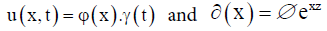then following the motion equation;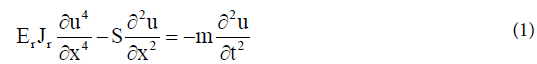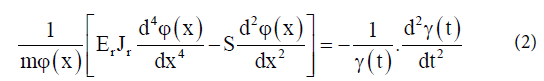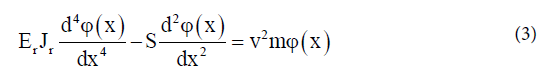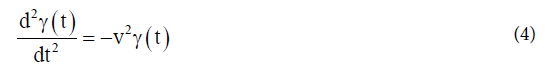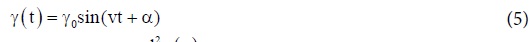Assuming that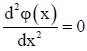when x=0 and l=x then one gets a partial resonance frequency. Where the resonance frequency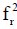relates to the vibration modes causing uncertainties and expressed as;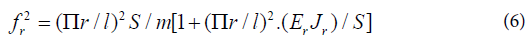This equation can be used to calculate bending stiffness of the transmission line. A value of this frequency could then be useful in line sag estimation. The future expansion of the transmission networks will also require analysis on bending limitations of the line. This can be done by looking at the lowest point of the conductor.

The estimatimated low point y(x), of the conductor defined as catenary curve (Figure 1).

Height of the conductor above this low point is then given by;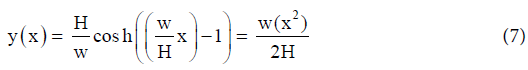For a level span, the low point is in the center, and the line sag, D, is found by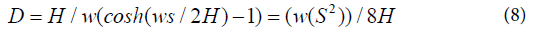The end of the level span, conductor tension T, is equal to the horizontal component plus the conductor weight per unit length,w , multiplied by the sag, D as shown in equation (9).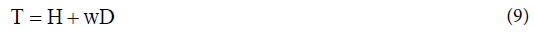Considering the conductor length from the low point of the catenary from either direction of the sag on the catenary curve can be obtained as follows: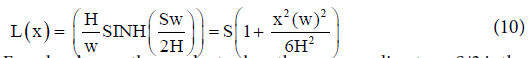For a level span, the conductor length corresponding to x=S/2 is the half of the total conductor length and the total length, L, is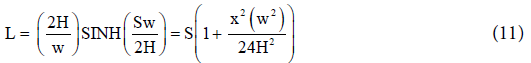Substituting parabolic equation of the sag one gets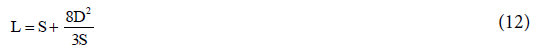Conductor slack is the difference between the conductor length, L, and the span length, S. The parabolic equations for slack may be found by combining the preceding parabolic equations for conductor length, L and sag, D .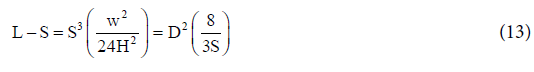A conductor slack in a span can contribute to the changes in conductor sag, such that: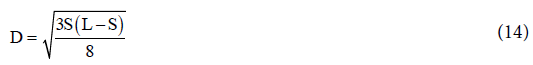For inclined spans, they may be analyzed using essentially the same equations that are used for equally levelled spans. The catenary equation for the conductor height above the low point in the span is the same. For the span, we consider two separate sections, on to the right of the low point and the other to the left of the low point. The shape of the catenary relative to the low point is unaffected by the difference in suspension point elevation. In each direction from the low point, the conductor elevation relative to the low point is [4,5].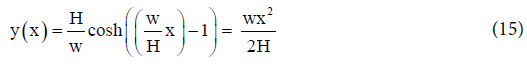The horizontal distance XL from the left support point to the low point in the catenary is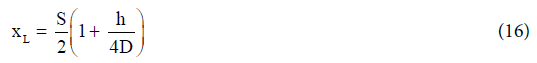The horizontal distance, XR, from the right support point to the low point of the catenary is :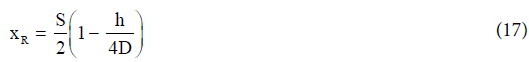From the inclined catenary span S1 is the straight-line distance between supporting points, S is the horizontal distance between supportsh is the vertical distance between support points, D is the sag measured vertically from a line through the points of conductor support to a line tangent to the conductor .

The midpoint sag Dis approximately equal to the sag in a horizontal span equal to the length to the inclined span. Knowing the horizontal distance from the low point to the support point in each direction, the preceding equations for y(x), L, D, and T can be applied to each side of the inclined span. The total length in the inclined span is equal to the sum of the lengths in XR and XL sub-span sections [4,6,7].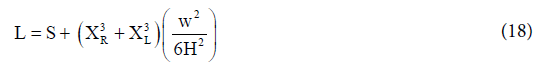View of a position vector for conductor

When we review the position of a stretched conductor as presented by authors [8,9], in the form of a position vector P(l, t) = (X(l, t), Y(l, t)) with corresponding tension vector

T(l, t) = T(l, t) (cosψ, sinψ),               (19)

where ø is the positively oriented angle between the cable tangent and the horizontal.

Tension vector is tangent to the cable or the conductor because of the assumed negligible bending stiffness. Although this might not always be the case, we agree with the authors [2,4,9], that the conductor element stretched under tension is represented as (dx2+dy2).11/2 (Figure 2).

When a small cable element is considered, dl. Due to gravity, cable tension, and inertial forces, this element is stretched however the mass remain the same. A conductor element is elongated in proportion to tension, according to Hooke’s law, such that :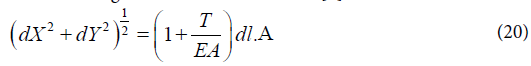According to a Newton’s law, the internal tension and the external gravity forces are in equilibrium with the inertial forces, such that;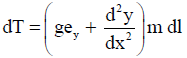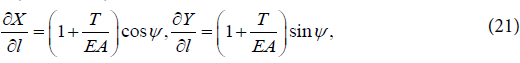The equation result in the limit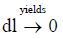are given in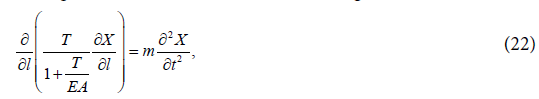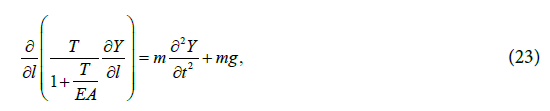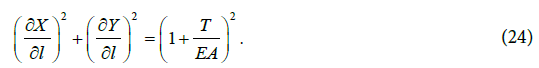For the boundary and coupling conditions in Figure 3,

Where L=0 and L=NL. For a fixed support

X = 0,Y = 0,(l = 0),

At suspension string :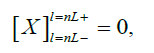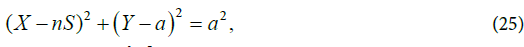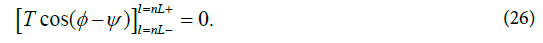Where ϕ is the angle of the supension string.

This approach helps to understand the problem of sag more clearly and the behavior of the transmission line under galloping conditions. Hence it helps to reduce various problem parameters in to a single clear problem.

Dynamic simulation

Computer program application to model the time varying behaviour of a system or in particular a transmission line, when it is described by ordinary differential equations or partial differential equations is known to be dynamic simulation.When bounding uncertainty is considered in dynamic simulation, correct parameter values is unlikely known. It is common for the range of a parameter value to be considered. A statistical distribution could then be estimated or predicted.

For instance we can assume that parameters are uniformly distributed between some lower and upper bounds. Alternatively, there may be sufficient data to assume a normal distribution with some mean and variance [10-13]. Simulation of large data sets is not accurate to predict uncertainties, since parameters are mostly based on assumptions.

The well know Monte-Carlo and Simulink technique is mostly used to quantify the uncertainty in a trajectory [14-16].When other transmission equipment is taken into consideration, such as transformers, the load time constant TP and the transformer tapping delay Ttap should be carefully looked after. This method results in errors occur due to uncertainties.

Interval mathematical approach

Interval mathematics use interval numbers instead of ordinary single point numbers. This interval number is defined as an ordered pair of real numbers representing the lower and upper bounds of the parameter range [17-19]. If the interval numbers are given as [a, b] and [c, d] then the following laws can be adapted for calculation;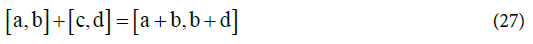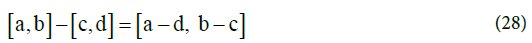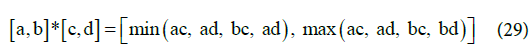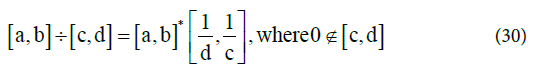Traditionally power transmission is performed using two or more conductors for single-phase or multiphase systems. A problem appears in three-phase lines as a result of distribution of the phases over the length of the line .

Positions of phase a, b, and c lines change in a cycle that puts each phase in three possible positions over the line span. This cycle is to ensure that each phase experiences an equivalent amount of flux linkage. Thus, the average inductance of each of the phases will be equal.

If all three conductors have the same radius r, they will have the same inductance L: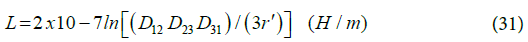Where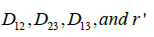are defined as follows:

D12 is the distance in meters between phase conductors 1 and 2, D23 is the distance in meters between phase conductors 2 and 3, and D13 is the distance in meters between phase conductors 1 and 3.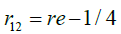which is the radius in meters the accounts for internal inductance .

Although the calculations of uncertainties can be performed with interval mathematics, the variation of conductor length during temperature changes and loads from rain, snow or ice needs to be taken into consideration; however it is hard to be known especially in the real time base.

Synchronized-phasor measurements

The network, Figure 4 represents line parameter estimation for sending and receiving end voltages and currents.

Where,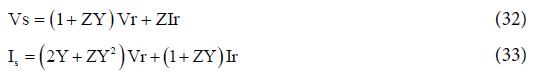Where Vs and Vr are the sending and receiving end voltages respectively. Is and Ir are sending and receiving end currents. Z and Yare impedance and admittance of the network.

Therefore,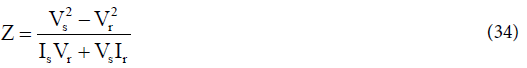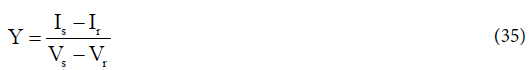Synchronization angle δ is used to synchronize the voltage phasors at both the terminals. Using the synchronization angle δ, the phasors at the bus i with respect to global positioning system (GPS) reference can be defined as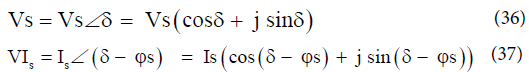Where φs is the power factor angle. Using a specified maximum measurement uncertainty, bounds of the line parameters can be calculated [19,20]. Using the values of the maximum measurement uncertainties, the standard uncertainties in the measurements can be calculated as;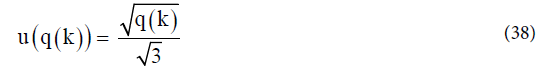Where, uq(k) is the maximum specified uncertainty specified by the manufacturer in the measurement of the parameter q(k).

The standard uncertainties in the power factor angle φs and the synchronization angle δ can be calculated: [20,21].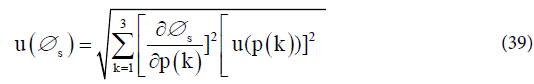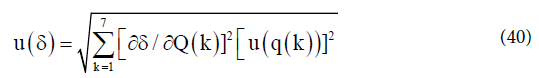Where φs, is the power factor which is determined using the reactive power Qs . P(k) is the phase current. The lagging and leading power factor can be differentiated by the sign of Qs. The power factor is lagging if the sign of Qs is –ve and it is leading if Qs is +ve.

The standard uncertainty in the power factor angle φs and the synchronization angle δ can be calculated by  classical uncertainty propagation theory.

Global positioning system

This is piloted based on a constellation of 24 satellites, which uses the Navigation satellite Timing and Ranging (NAVSTAR) developed, launched, and maintained by the United States government [23-29]. This method relies on accurate time-pulsed radio signals in the order of nanoseconds from high altitude Earth orbiting satellites of about 11 000 nautical miles, with the satellites acting as precise reference points. These signals are transmitted on two carrier frequencies known as the L1 and L2 frequencies. The L1 carrier is 1.5754GHz and carries a pseudo-random code (PRC) and the status message of the satellites.

There is a potential in this technology, although there are some errors contributing to estimates. The differential GPS is generally used in order to decrease the selective availability errors. The accuracy of the direct instrumentation of overhead power line conductor sag measurement is about 19.6 cm range and 70% of the time [30-35].

This technique is promising, however the challenge such as electromagnetic interference (EMI) from the phase conductors is questionable [36,37].

Detection via image processing

There are some optimized edge detectors in image processing, such as canny edge detectors and infinite symmetric exponential filter. These detectors follow different algorithms such as for canny edge detection, it is represented as follows [38,39]:

2. Convolve a 1D Gaussian mask with I.

3. Create a 1D mask for the first derivative of the Gaussian in the x and y directions.

4. Convolve I with G along the rows to obtain Ix, and down the columns to obtain Iy.

5. Convolve Ix with Gx to have Ix’, and Iy with Gy to have Iy’.

6. Find the magnitude of the result at each pixel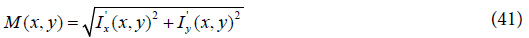The Infinite Symmetric Exponential Filter uses another optimization function to find the edge in an image this function can be written as :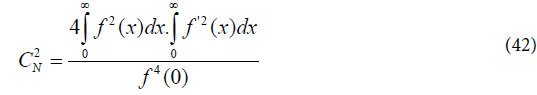The function CN is minimized with an optimal smoothing filter for an edge detector, which results in an infinite symmetric exponential filter, as follows :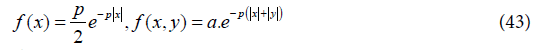The filter in the infinite symmetric exponential filter edge detector is presented as one dimensional recursive filter. P is the probabilitic transition , x and y are horizontal and vertical deravetieves By presuming the 2D-filter function real continuous, it is given by ;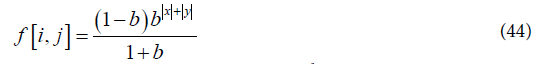In some instances, image processing techniques use automatic image analysis technique for extracting information from the line insulators. No uncertanty predictions can be evident with this technique.

#### Conclusion

The current power system is ideally exposed to different environmental factors which result in uncertainty problems. The methods discussed in this paper mostly do the determination of line parameters by means of calculations. Even though calculations are somehow accurate, errors do occur in the life of existing lines due to unpredicted temperature boundaries, wind forces, load from snow or ice, etc. The challenge still remains to develop a technique for real-time uncertainty modeling tool, which can be used to detect live transmission line conductor directly, for further accurate measurements and studies. GPS could be more valuable in future if the EMI challenge is conquered. The advancement in research for other technologies based on electromagnetic field theory is needed to address these limitations. Interval mathematics is more versatile compared to other methods such as synch phasor technique. The unknown variable can be stretched while bounded and it has shown to be faster than Monte Carlo methods in solving alternating current network problems.

#### References

Select your language of interest to view the total content in your interested language

### Article Usage

• Total views: 11096
• [From(publication date):
September-2016 - Jan 24, 2020]
• Breakdown by view type
• HTML page views : 10805Can't read the image? click here to refresh